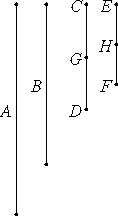# Proposition 20

The least numbers of those which have the same ratio with them measure those which have the same ratio with them the same number of times; the greater the greater; and the less the less.

Let CD and EF be the least numbers of those which have the same ratio with A and B.

I say that CD measures A the same number of times that EF measures B.Now CD is not parts of A. If possible, let it be so. Therefore EF is also the same parts of B that CD is of A.

Therefore there are as many parts of B in EF are there are parts of A in CD.

Divide CD into the parts of A, namely CG and GD, and divide EF into the parts of B, namely EH and HF. Thus the multitude of CG and GD equals the multitude of EH and HF.

Now, since the numbers CG and GD equal one another, and the numbers EH and HF also equal one another, while the multitude of CG and GD equals the multitude of EH and HF, therefore CG is to EH as GD is to HF.

VII.12

Since one of the antecedents is to one of the consequents as the sum of the antecedents is to the sum of the consequents, therefore CG is to EH as CD is to EF.

VII.4

Therefore CG and EH are in the same ratio with CD and EF, being less than they, which is impossible, for by hypothesis CD and EF are the least numbers of those which have the same ratio with them. Therefore CD is not parts of A, therefore it is a part of it.

And EF is the same part of B that CD is of A, therefore CD measures A the same number of times that EF measures B.

Therefore, the least numbers of those which have the same ratio with them measure those which have the same ratio with them the same number of times; the greater the greater; and the less the less.

Q.E.D.

## Guide

This proposition says that given a ratio a : b, if c : d is the same ratio and the least among all those ratios with the same ratio, then, first of all, c divides a, and d divides b, but also, c divides a the same number of times that d divides b. For example, the ratio 91 : 132 is the same ratio as 7 : 11, which is least among all the ratios equal to 91 : 132, that is to say 91 : 132 reduces to 7 : 11 in lowest terms, therefore 7 divides 91 the same number of times that 11 divides 132, namely, 13 times.

The proof goes along like this. Suppose a : b reduces to c : e in lowest terms. In order to show that c divides a, assume that it doesn’t, assume that c = (m/n)a. Since a : b is the same ratio as c : e, therefore e = (m/n)d. But then c/m = (1/n)a, and e/m = (1/n)b. Therefore c/m : e/m is the same ratio as a : b, which shows that c : e is not in lowest terms, a contradiction. Therefore c does divide a, and e divides b the same number of times.

#### Use of this proposition

This proposition is used frequently in Books VII through IX starting with the next proposition.